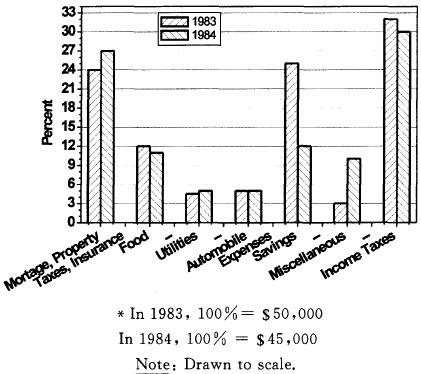On July 1st the ratio of men to women in Club X was 9 to 20. During the month, 2 additional men and 2 additional women joined the club, and no members dropped out.

Column A Column B

The ratio of men to women in

Club X at the end of July 1/2

A．if the quantity in Column A is greater

B．if the quantity in Column B is greater

C．if the two quantity are equal

D．if the relationship cannot be determined from the information given

• If x is percent more than y, then y is what percent less than x ?A.9%B.10%C.11%D.12%E.15%

If x ispercent more than y, then y is what percent less than x ?

A．9%

B．10%

C．11%

D．12%

E．15%

• FAMILY X'S EXPENDITURES AS A PERCENT OF ITS GROSS ANNUAL INCOME *Family X's gross income i

FAMILY X'S EXPENDITURES AS A PERCENT OF ITS GROSS ANNUAL INCOME *Family X's gross income is the sum of Mr. X's income and Mrs. X's income. In 1983 Mr. and Mrs. X each had an income of \$25,000. If Mr. X's income increased by 10 percent from 1983 to 1984, by what percent did Mrs. X's income decrease for the same period?

A．10%

B．15%

C．20%

D．25%

E．30%